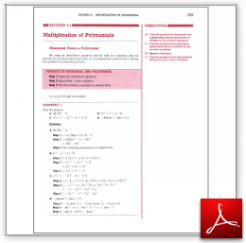Algebra Math Book - Introductory Algebra Thank you for your continual support.

 The power of mathematics rests in the logic of thinking.ID: Sec4-5
Description: Monomial Time a Polynomial-Multiplying Binomials-FOIL-Squaring a Binomial
Price: 1.99

# Algebra Math book - Introductory Algebra - Chapter 4 - Section 5 - Multiplication of Polynomials

## Section 4.5 - Objectives

10.  Find the product of a monomial and a polynomial where each factor is written in one or more variables.

11.  Find the product of two binomials where each factor is written in one or more variables.

12.  Square a binomial.

13.  Find the product of two polynomials written in one or more variables.

Navigate to
Previous Section or Next Section
or Chapter 4 Details or Other Chapters

This section of my algebra math book, Introductory Algebra, also includes in the download:

• Cover Sheet